# Battery Cycle Life Prediction Using Deep Learning

Lithium-ion battery cycle life prediction using a physics-based modelling approach is very complex due to varying operating conditions and significant device variability even with batteries from the same manufacturer. Further, every battery ages differently depending on usage and conditions during manufacturing. In this example, we illustrate the use of deep learning technique for estimating the remaining cycles of a fast charging lithium-ion battery. Data representing the full lifecycle of the batteries is used to train a 2D Convolution Neural Network based architecture and this trained network is used to estimate the remaining cycle life of new batteries.

#### Dataset

The dataset contains measurements from 40 lithium-ion cells with nominal capacity of 1.1 Ah and a nominal voltage of 3.3 V under various charge and discharge profiles. Each battery is charged and discharged, according to one of many predetermined policies, until the battery reaches 80% of its original capacity. The number of cycles until this state is reached is called the battery cycle life. This number varies broadly between 150 and 2300 cycles as seen in the histogram of the data used for this example.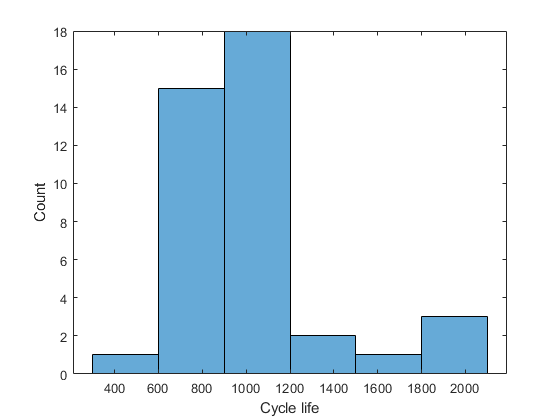The full dataset containing measurements from 124 cells can be accessed here  with detailed description here . This example uses a reduced dataset containing measurements from 40 cells only to make it easier to download and to run this example. Data for each battery is stored in a structure, which includes the following information:

• Data collected within a cycle: Current, voltage, temperature, capacity, differential discharge capacity

Load the data from the MathWorks supportfiles site (this is a large dataset, ~1.2GB).

```url = 'https://ssd.mathworks.com/supportfiles/predmaint/batterycyclelifeprediction/v2/batteryDischargeData.zip'; websave('batteryDischargeData.zip',url); unzip('batteryDischargeData.zip') load('batteryDischargeData');```

Visualize the data characteristics by creating a plot of current, voltage, and temperature measurements for one full cycle of the first battery in the data.

```battIndx = 1; cycleIndx = 1; batteryMeasurements = table(batteryDischargeData(battIndx).cycles(cycleIndx).I,batteryDischargeData(battIndx).cycles(cycleIndx).V,... batteryDischargeData(battIndx).cycles(cycleIndx).T, batteryDischargeData(battIndx).cycles(cycleIndx).Qd); stackedplot(batteryMeasurements, "Title","Measurements over one cycle",... "DisplayLabels", ["Current (A)","Voltage(V)","Temperature(C)","DischargeCapacity(Ah)"], ... "Xlabel", "Sample Index");```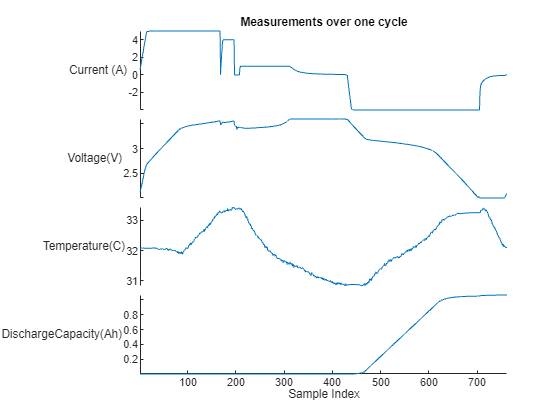In the preceding plot, positive current indicates a charging process while negative current indicates a discharge operation. The battery is fully charged when it reaches 3.6V and fully discharged when it reaches 2V. Further, the batteries are subjected to different fast charging policies in this dataset to understand their degradation profile across time and load.

### Extract Battery Discharge Measurements

Since all batteries have different charging policies but identical discharge voltage range, you use only the discharge portions of the signals in this example. Extract the measurements corresponding to the discharge portion of the cycle using the `hExtractDischargeData` helper function. Plot the discharge data for the first cycle of the first battery.

```dischargeData = hExtractDischargeData(batteryDischargeData); batteryMeasurements = table(dischargeData{battIndx}.Vd{cycleIndx},dischargeData{battIndx}.Td{cycleIndx},... dischargeData{battIndx}.QdClipped{cycleIndx}); stackedplot(batteryMeasurements, "Title","Measurements over one cycle",... "DisplayLabels", ["Voltage(V)","Temperature(C)","DischargeCapacity(Ah)"],... "Xlabel", "Sample Index");```Since the batteries in this data set are tested with different charging policies, some cycles are completed sooner than others. Therefore, cycle time cannot be used to compare charge and temperature across batteries. The voltage range is used as the reference instead of time because the discharge time varies based on the connected load and the health of the batteries. The charge and temperature measurements are then interpolated over this voltage range. Use `hLinearInterpolation `function to interpolate voltage, temperature and discharge capacity measurements onto a uniformly sampled 900 point voltage range between 3.6V and 2V. The interpolated data is returned as a 30x30 array for each measurement to form a 2D representation for each battery discharge cycle. Note that reshaping the `900x1` vector to a `30x30` matrix leads to the convolutional network searching for spatial relation between each column of the matrix. This example assumes that such a relationship might exist across the various cycles and attempts to leverage it if present. The image below shows the temperature and voltage data for a single cycle of a cell being interpolated to 900 points and then being reshaped to a 30x30. The 2D representation the measurement of each cycle converts the sensor measurements into an image format for the CNN layers.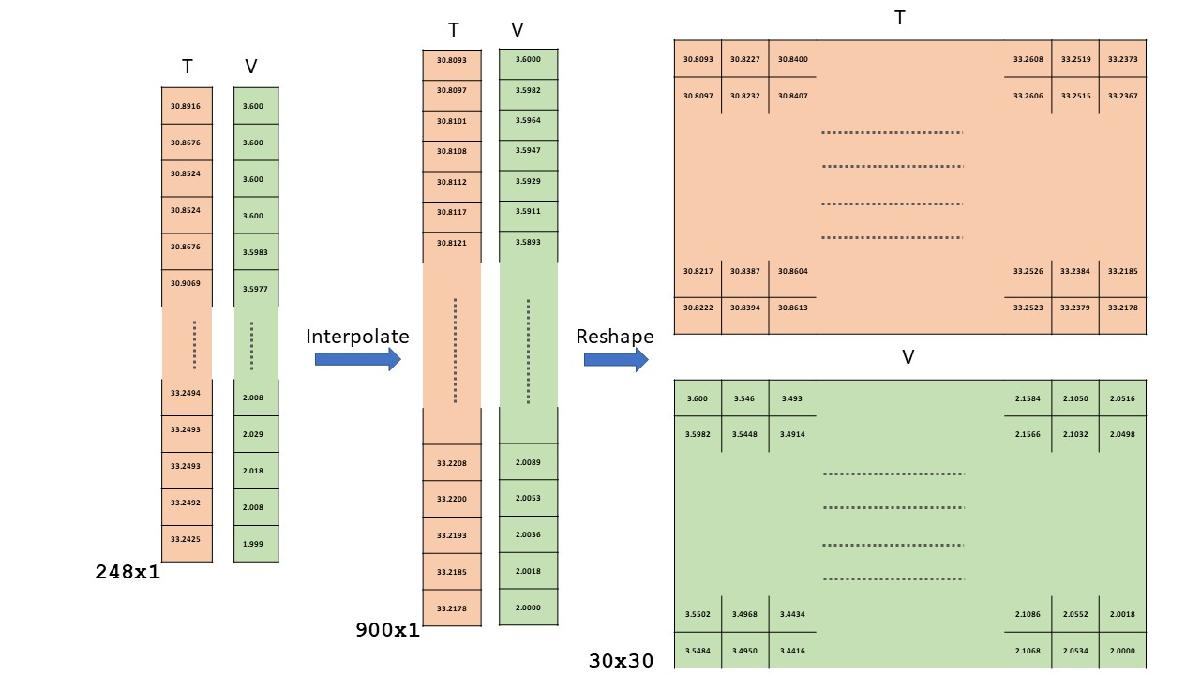`[VInterpol,TInterpol,QdInterpol] = hLinearInterpolation(dischargeData);`

Plot the interpolated temperature and discharge capacity as a function of voltage.

```figure yyaxis left plot(reshape(VInterpol{1}{1}, 900, 1),reshape(TInterpol{1}{1},900,1)) title('Measurements as a function of Voltage') ylabel('Temperature') xlabel('Voltage') yyaxis right plot(reshape(VInterpol{1}{1},900,1),reshape(QdInterpol{1}{1},900,1)) ylabel('Discharge Capacity')```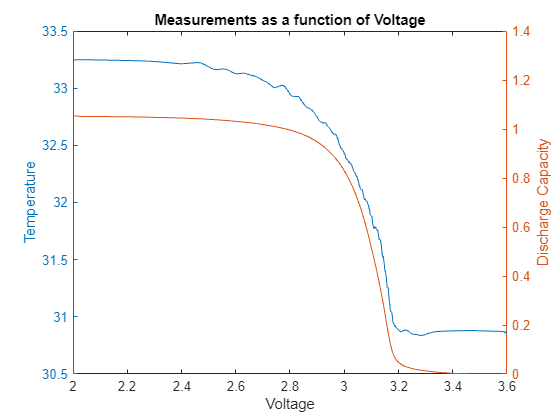For the 2D Convolution Neural Network layers in the deep network, the 30x30 matrix of interpolated voltage, discharge capacity and temperature are reshaped to a form a 30x30x3 matrix for each cycle. This is like the RGB channels of an image. To minimize the range of the estimated remaining cycles, the expected output signal is normalized by dividing by 2000 (the maximum life of the battery in the data). The data from 30 batteries is used for training, 5 batteries for validation and 5 batteries for testing of the deep neural network. Use the `hreshapeData` helper function to create the 30x30x3 dataset for each cycle. This function outputs the measurement data (`trainData)` and the RUL data (`trainRulData)` to use as labels for each case.

```testBatteryIndex = 2:8:40; valBatteryIndex = 1:8:40; trainBatteryIndex = setdiff(1:40,[2:8:40 1:8:40]); [trainData,trainRulData] = hreshapeData(VInterpol(trainBatteryIndex), ... TInterpol(trainBatteryIndex),QdInterpol(trainBatteryIndex)); [valData,ValRulData] = hreshapeData(VInterpol(valBatteryIndex), ... TInterpol(valBatteryIndex),QdInterpol(valBatteryIndex)); [testData,testRulData] = hreshapeData(VInterpol(testBatteryIndex), ... TInterpol(testBatteryIndex),QdInterpol(testBatteryIndex)); fprintf('Size of reshaped matrix of interpolated measurement data:%dx%dx%dx%d\n', ... size(trainData))```
```Size of reshaped matrix of interpolated measurement data:30x30x3x30706 ```

### Define Network Architecture

Defining the architecture of convolutional neural network includes selecting the types of layers, selecting the number of layers, and tuning the hyperparameters until satisfactory performance is achieved against test data. In this section, the types of layers and number of layers are specified. To create your deep neural network structure, define a set of successive network layers. Use a network structure with the following layers:

• Image input layer — Treat the voltage, discharge capacity, temperature data as the three color channels of an input image and normalize the measurements to the range [0,1].

• 2D convolutional layers — Each of these layers applies sliding convolutional filters to the image input. This example uses four hidden convolutional layers. This number of layers, which was selected through trial and error, gives the best result while keeping a reasonable training time.

• Batch normalization layers — Each convolutional layer is followed by a batch normalization layer, which speeds up the training of the network and reduces the sensitivity to network initialization.

• ReLU layers — Each batch normalization layer is followed by a nonlinear activation function, which performs a threshold operation to each element of the input.

• Pooling layers — The first two batch ReLU layers are followed by pooling layers, which reduce the size of the feature map and remove redundant information, which reduces the number of parameters to be learned in subsequent layers.

• Drop-out layer — The final ReLU layer is followed by a dropout layer, which helps reduce overfitting in the network.

• Fully connected layer — The dropout layer is followed by a fully connected layer, which combines all of the learned features into a single input to the regression layer.

• Regression layer — Since the estimation of remaining useful life is a regression problem, the final output layer of the network is a regression layer.

```layers = [ imageInputLayer([30 30 3],"Normalization","rescale-zero-one") convolution2dLayer(3,8,"Padding","same") batchNormalizationLayer reluLayer maxPooling2dLayer(2,"Stride",2) convolution2dLayer(3,16,"Padding","same") batchNormalizationLayer reluLayer averagePooling2dLayer(2,'Stride',2) convolution2dLayer(3,32,'Padding','same') batchNormalizationLayer reluLayer convolution2dLayer(3,32,'Padding','same') batchNormalizationLayer reluLayer convolution2dLayer(3,32,'Padding','same') batchNormalizationLayer reluLayer dropoutLayer(0.5) fullyConnectedLayer(1) regressionLayer]; figure plot(layerGraph(layers))```### Define Network Hyperparameters and Train Network

In this section, define the hyperparameters for the network specified in the previous section. Selecting hyperparameters, such as the learning rate or batch size, is generally through trial and error with the goal of finding the best set for the selected network and the data set to achieve satisfactory performance from the network.

For this example, use the Adam (adaptive moment estimation) optimizer, which has a fast computation time and few parameters to tune. Configure the solver to:

• Use a mini-batch size of 256 observations.

• Train on the entire data set 50 times, which the number of training epochs.

• Shuffle the dataset before each epoch to improve convergence.

• Use a learning rate of 0.001, which achieves a good balance between convergence and overshooting.

• Validate the network periodically to identify when the network is overfitting the training data.

For more information on training options for the Adam solver, see `TrainingOptionsADAM`. The training hyperparameters used in this example were selected based using trial-and-error experimentation. You can adjust the parameters to further improve the training.

```miniBatchSize = 256; validationFrequency = 10*floor(numel(trainRulData)/miniBatchSize); options = trainingOptions("adam", ... "MaxEpochs",100, ... "MiniBatchSize",miniBatchSize, ... "Plots","training-progress", ... "Verbose",false, ... "Shuffle","every-epoch", ... "InitialLearnRate",0.001, ... "OutputNetwork","best-validation-loss", ... "ValidationData",{valData, ValRulData}, ... "ValidationFrequency",validationFrequency, ... "ValidationPatience",10, ... "ResetInputNormalization",false); rng("default") batteryNet = trainNetwork(trainData, trainRulData,layers,options);```### Evaluate Performance of Trained Model

Use the trained model to predict the remaining cycle life for `testData`. The values must be rescaled back to the original RUL range to make it easier to visualize the performance.

```yPredTest = predict(batteryNet,testData)*2000; testRulScaled = testRulData*2000;```

Compare the actual cycle life with the predicted cycle life using a scatter plot.

```figure; scatter(testRulScaled,yPredTest) hold on; refline(1,0); title("Predicted vs Actual Cycle Life") ylabel("Predicted cycle life"); xlabel("Actual cycle life");```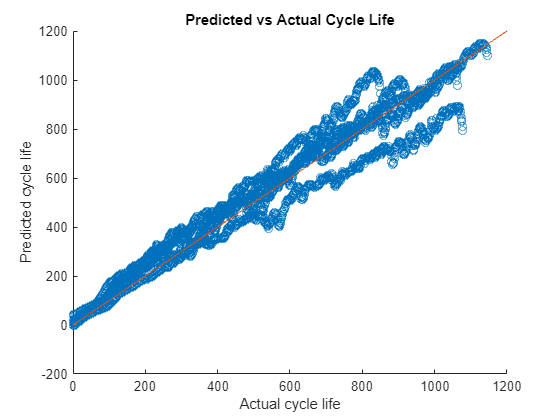Ideally, the scatter plot should have all data points along the diagonal with a narrow confidence band. However, in this example, there is a broader spread and different behaviors for different range of values in the scatter plot. In the scatter plot, there five distinct trends, one for each battery in the test data.

Across the five batteries, when the actual cycle life is small, the model is good at predicting the remaining useful life. This result implies that, as a battery gets closer to the end of its life, the model is good at predicting the remaining cycle life.

However, during the early part of a battery's life when the actual cycle life is larger, the model has greater uncertainty. The model also seems to generally overestimate the remaining cycle life during the initial period of a battery's life. To address these model characteristics, you can train the network using richer and larger data sets and experiment with the deep neural network architecture and its hyperparameters.

Compute the root mean squared error (RMSE) and the average percentage error of the predicted remaining cycle life.

```errTest = (yPredTest-testRulScaled); rmseTestModel = sqrt(mean(errTest.^2))```
```rmseTestModel = single 65.2403 ```
```n = numel(testRulScaled); nr = abs(testRulScaled - yPredTest); errVal = (1/n)*sum(nr./testRulScaled)*100```
```errVal = single 15.4083 ```

These performance metrics are relatively close to their equivalent values for when a linear regression model with regularization is used with custom features for estimating remaining cycle life, as shown in Battery Cycle Life Prediction From Initial Operation Data (Predictive Maintenance Toolbox). Note that in the machine learning based example only the first 100 cycles of data is used for estimating the remaining cycle life while in this example data from any cycle can be used. This result indicates that depending on the application and system requirements, either a machine learning or a deep learning approach can be used to estimate the remaining cycle life of batteries.

After tuning the model to a desired performance level, you can operationalize it to estimate remaining cycle life of batteries in use. To deploy a trained network to embedded hardware, generate C/C++, GPU, or HDL code. For more information, see Code Generation. To deploy the trained network to the cloud, choose the appropriate packaging option.

### Conclusion

This example shows how to use deep learning techniques for battery cycle life prediction based on measurements from 40 batteries. Raw sensor signals are directly used as inputs to train a deep neural network without any manual extraction of features. This model is used on test data for performance evaluation. Using measurements for the test data, the average percentage error is ~15%.

### Helper Functions

```function [dischargeData] = hExtractDischargeData(data) % HEXTRACTDISCHARGEDATA Extract measurements corresponding to discharge % portion of cycle dischargeData = cell(1, size(data, 2)); % For each battery in the data (which has many charge discharge cycles) for iBattery = 1:size(data,2) timeSeriesTable = struct2table(data(iBattery).cycles); % Keep only the data related to discharge [ between 3.6V and 2 V) clipIdxFun1 = @(x) {find(x{1,1}>=3.6,1,"last")}; clipIdxFun2 = @(x) {find(x{1,1}<=2.00,1,"first")}; clipIdx1 = rowfun(clipIdxFun1,timeSeriesTable,"InputVariables","V",... "OutputVariableNames","clipIdx1"); clipIdx2 = rowfun(clipIdxFun2,timeSeriesTable,"InputVariables","V",... "OutputVariableNames","clipIdx2"); timeSeriesTable = [timeSeriesTable clipIdx1 clipIdx2]; clipSignals = @(x,y,z) {smoothdata(x(y:z),"movmean",3)}; % Extract Voltage Vd = rowfun(clipSignals,timeSeriesTable,"InputVariables",... ["V","clipIdx1","clipIdx2"],"OutputVariableNames","Vd",... "ExtractCellContents",true); % Extract Temperature Td = rowfun(clipSignals,timeSeriesTable,"InputVariables",... ["T","clipIdx1","clipIdx2"],"OutputVariableNames","Td",... "ExtractCellContents",true); % Extract Discharge Capacity QdClipped = rowfun(clipSignals,timeSeriesTable,"InputVariables",... ["Qd","clipIdx1","clipIdx2"],"OutputVariableNames","QdClipped",... "ExtractCellContents",true); dischargeData{iBattery} = [Vd Td QdClipped]; end end function [Vdlin, Tdlin, Qdlin] = hLinearInterpolation(dischargeData) % HLINEARINTERPOLATION Interpolate on the voltage range of 2V to 3.6V % linear interpolation onto 900 points between the two voltages and the % data is then reshaped into a 30x30 matrix Vdlin = cellfun(@(x)rowfun(@hLinInterp,x,"InputVariables",["Vd","Vd"],... "OutputVariableNames","Vdlin","OutputFormat","cell"), dischargeData, ... 'UniformOutput', false); Tdlin = cellfun(@(x)rowfun(@hLinInterp,x,"InputVariables",["Vd","Td"],... "OutputVariableNames","Tdlin","OutputFormat","cell"), dischargeData, ... 'UniformOutput', false); Qdlin = cellfun(@(x)rowfun(@hLinInterp,x,"InputVariables",["Vd","QdClipped"],... "OutputVariableNames","Qdlin","OutputFormat","cell"), dischargeData, ... 'UniformOutput', false); end function xInterpolated = hLinInterp(volt,x) % HLININTERP Function to linearly interpolate data for battery voltage discharge range volt = volt{1,1}; x = x{1,1}; % Set seed for consistent results rng("default"); % Linearly interpolate voltage range 3.6 to 2. voltRange = linspace(3.6,2,900); [~, ia, ~] = unique(volt,'sorted'); f = griddedInterpolant(volt(ia),x(ia)); xInterpolated= reshape(f(voltRange)',[30,30]); end function [signalData, rul] = hreshapeData(VInterpol, TInterpol, QdInterpol) % HRESHAPEDATA Arrange the data as 30x30x3 - where each 30x30 is the 900 point % interpolated version for a single discharge and 3 is for V, Q, T for i =1:numel(VInterpol) VData = VInterpol{i}; TData = TInterpol{i}; QdData = QdInterpol{i}; predictor = zeros(30,30,3,size(VData,1)); for j = 1: size(VData,1) temp(:,:,1) = VData{j,1}; temp(:,:,2) = QdData{j,1}; temp(:,:,3) = TData{j,1}; predictor(:,:,:,j) = temp; end maxBatteryLife = 2000; % Used for scaling output numCycles = size(VData,1); cycle = (1:numCycles)'; rulBattery = (numCycles+1 - cycle)/maxBatteryLife; if i == 1 signalData = predictor; rul = rulBattery; else signalData = cat(4,signalData,predictor); rul = [rul; rulBattery]; end end end```

#### References

 Severson, K.A., Attia, P.M., Jin, N. et al. "Data-driven prediction of battery cycle life before capacity degradation." Nat Energy 4, 383–391 (2019). https://doi.org/10.1038/s41560-019-0356-8